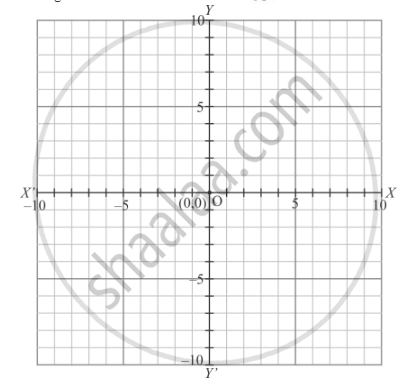# The Measure of the Angle Between the Coordinate Axes is - Mathematics

MCQ

The measure of the angle between the coordinate axes is

• 90°

• 180°

• 360°

#### Solution

As we know that x−axis and y−axis intersect to each other at point O and perpendicular to each other. So, the angle between the coordinate axes is 90° .Concept: Coordinate Geometry
Is there an error in this question or solution?

#### APPEARS IN

RD Sharma Mathematics for Class 9
Chapter 8 Co-ordinate Geometry
Exercise 8.2 | Q 3 | Page 7

Share